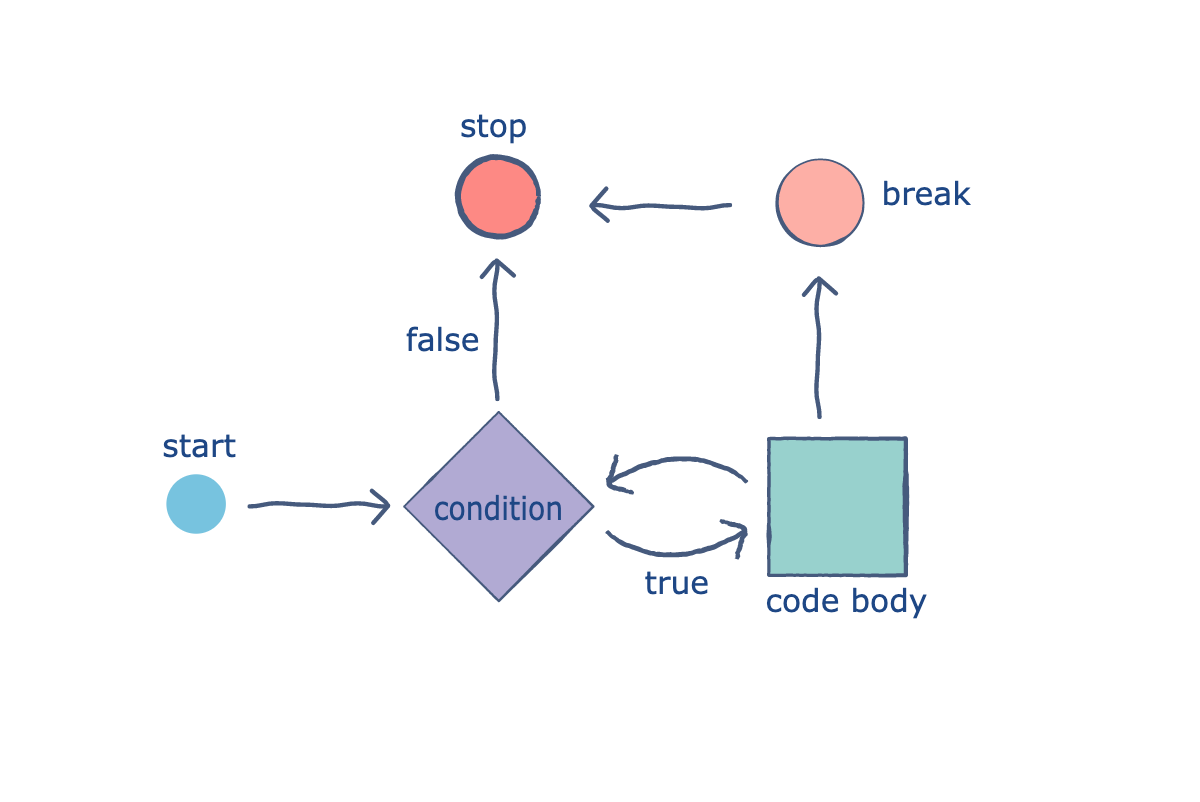Related Tags

break
loops
php

# The PHP break statementEducative Answers Team

A break statement is an intentional interruption that prevents the loop from running. Sometimes a situation arises that makes us want to exit from a loop immediately without waiting to get back to the conditional statement.

The keyword break ends execution of the current for, foreach, while, or do while loops.

When the keyword break is executed inside a loop, the control is automatically passed on to the first statement outside of the loop.A break is usually associated with the if statement.

## Code

In the following example, we test the value of $sum; if it becomes greater than 1300, the break statement terminates the execution of the code. As the echo statement is the first statement outside of the loop, it will print the current value of $sum.

Take a look at the code below:

<?php
$array1=array(100, 1100, 200, 400, 900);$x1=0;
$sum=0; while ($x1<=4)
{
if ($sum>1300) { break; }$sum = $sum+$array1[$x1];$x1=$x1+1; } echo$sum;
echo "\n";
echo "The loop ran ", \$x1, " times";
?>


After adding the first three values the sum became 1400, the break statement was executed, and the loop was terminated. This is why the loop only ran three times.

RELATED TAGS

break
loops
php